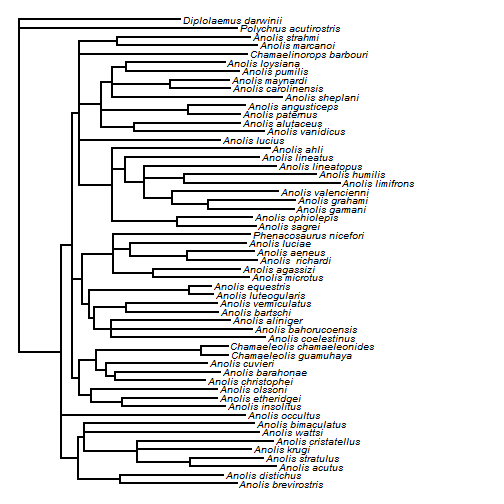## Monday, October 12, 2015

### Re-write of internally used function phyloDesign

I have totally re-written the internally used function, `phyloDesign`, which computes a design matrix for least-squares phylogeny estimation in the phytools function `optim.phylo.ls`, which does unweighted least-squares phylogeny estimation from a distance matrix.

Here's a simple demo using a DNA dataset from Jackman et al. (1999; Syst. Biol.).

``````library(devtools)
install_github("liamrevell/phytools",quiet=TRUE)
``````
``````packageVersion("phytools")
``````
``````##  '0.5.3'
``````
``````library(phytools)
dna<-as.DNAbin(dna)
dna
``````
``````## 55 DNA sequences in binary format stored in a list.
##
## All sequences of same length: 1456
##
## Labels: Diplolaemus_darwinii Polychrus_acutirostris Anolis_acutus Anolis_cristatellus Anolis_krugi Anolis_stratulus ...
##
## Base composition:
##     a     c     g     t
## 0.339 0.261 0.118 0.281
``````
``````## compute distance matrix
D<-dist.dna(dna)
## estimate tree, using NJ as a starting tree:
ls.fit<-optim.phylo.ls(D,phangorn::NJ(D))
``````
``````## 1 set(s) of nearest neighbor interchanges. best Q so far = 0.187309346
## 2 set(s) of nearest neighbor interchanges. best Q so far = 0.185881266
## 3 set(s) of nearest neighbor interchanges. best Q so far = 0.1847435677
## 4 set(s) of nearest neighbor interchanges. best Q so far = 0.1839579959
## 5 set(s) of nearest neighbor interchanges. best Q so far = 0.1835163321
## 6 set(s) of nearest neighbor interchanges. best Q so far = 0.1830769343
## 7 set(s) of nearest neighbor interchanges. best Q so far = 0.1827496093
## 8 set(s) of nearest neighbor interchanges. best Q so far = 0.18261176
## 9 set(s) of nearest neighbor interchanges. best Q so far = 0.1823425143
## 10 set(s) of nearest neighbor interchanges. best Q so far = 0.1821113405
## 11 set(s) of nearest neighbor interchanges. best Q so far = 0.1819861917
## 12 set(s) of nearest neighbor interchanges. best Q so far = 0.1819386781
## 13 set(s) of nearest neighbor interchanges. best Q so far = 0.1819014877
## 14 set(s) of nearest neighbor interchanges. best Q so far = 0.1814029139
## 15 set(s) of nearest neighbor interchanges. best Q so far = 0.1813641191
## 16 set(s) of nearest neighbor interchanges. best Q so far = 0.180996378
## best Q score of 0.180996378 found after 16 nearest neighbor interchange(s).
``````
``````plotTree(root(ls.fit,outgroup="Diplolaemus_darwinii",resolve.root=TRUE),
fsize=0.8,ftype="i")
``````It's hard to summarize where the speed-up in `phyloDesign` (which is many-fold for reasonable sized trees) comes from. The original version was written with loops & logical statements. The new version with `apply` family functions and set comparisons. Basically, I'm a bit better at R programming nowadays than I was five years ago when I wrote the earlier version of this function. (But still not all that good….)

That's it!